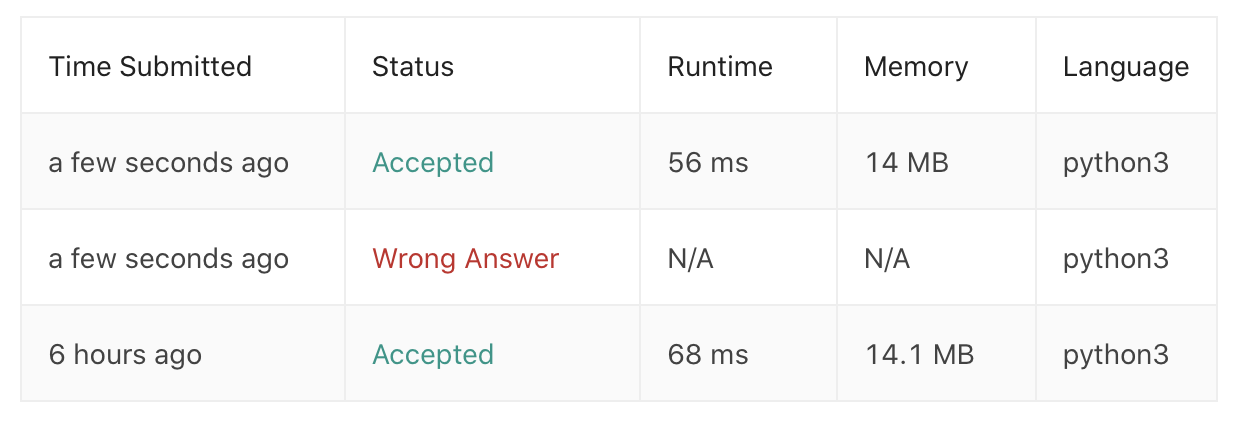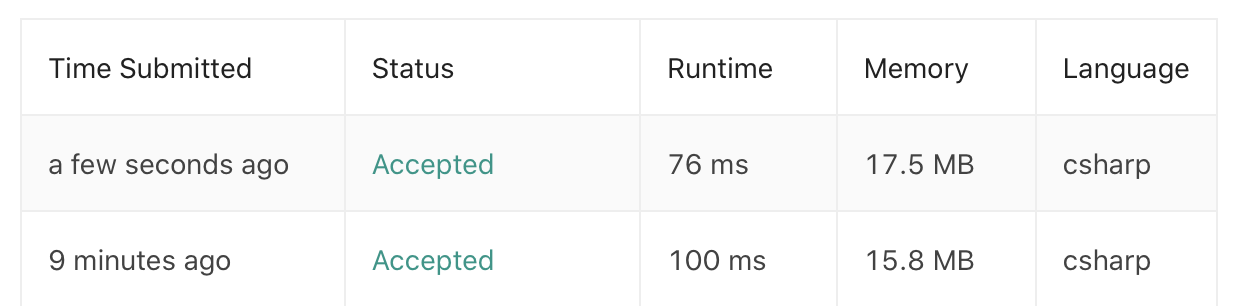LeetCode | 4. Palindrome Number 回文数PuranPalindrome Number 是 LeetCode 算法题库中的第九道题，难度为 Easy，题目地址为：https://leetcode.com/problems/palindrome-number/

Input: 121
Output: true
﻿
Input: -121
Output: false

﻿

2. 解题思路

1. 将整数转换为字符串后来比对，这种方法最为简单。

2. 借用堆栈的 Pop/Push方法，对整数本身进行处理，来得到反转后的整数并进行比较。

﻿

4. 代码

Python 实现

• 将整数转为字符串

class Solution:
def isPalindrome(self, x: int) -> bool:
x_reverse = str(x)[::-1]
if (x_reverse == str(x)):
return True
else:
return False

﻿

• 借用堆栈的 Pop/Push 方法

class Solution:
def isPalindrome(self, x: int) -> bool:
if x >= 0:
x_reverse = 0
x_origin = x
while (x != 0):
pop = x % 10
x = x // 10
x_reverse = int(x_reverse * 10 + pop)
if (x_reverse == x_origin):
return True
else:
return False
else:
return False

﻿﻿

C# 实现

• ‍将整数转为字符串

public class Solution {
public bool IsPalindrome(int x) {
string x_str = x.ToString();
char[] x_char = x_str.ToCharArray();
Array.Reverse(x_char);
string x_reverse = new string(x_char);
if (x_reverse == x_str)
{
return true;
}
else
{
return false;
}
}
}

﻿

• ‍借用堆栈的 Pop/Push 方法

public class Solution {
public bool IsPalindrome(int x) {
int x_reverse = 0;
int x_origin = x;
if (x >= 0)
{
while (x != 0)
{
int pop = x % 10;
x = x / 10;
x_reverse = 10 * x_reverse + pop;
}
if (x_reverse == x_origin)
{
return true;
}
else
{
return false;
}
}
else
{
return false;
}
}
}

﻿﻿Puran

GIS从业者，正在往开发的路上小跑。 2018.03.29 加入

评论